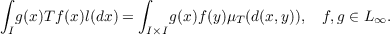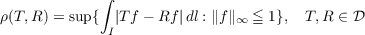#### Vol. 26, No. 3, 1968

 Download this articleFor screen For printingRecent Issues Vol. 325: 1  2 Vol. 324: 1  2 Vol. 323: 1  2 Vol. 322: 1  2 Vol. 321: 1  2 Vol. 320: 1  2 Vol. 319: 1  2 Vol. 318: 1  2Online Archive Volume: Issue:The Journal Subscriptions Editorial Board Officers Contacts Submission Guidelines Submission Form Policies for Authors ISSN: 1945-5844 (e-only) ISSN: 0030-8730 (print) Special Issues Author Index To Appear Other MSP Journals
Uniform approximation of doubly stochastic operators

### Choo Whan Kim

Vol. 26 (1968), No. 3, 515–527
##### Abstract

Let L = L[0,1] be the real Banach space of essentially bounded Lebesgue measurable functions on the unit interval I = [0,1] with the essential sup-norm. A positive linear operator T : LL is called doubly stochastic if (1) T1 = 1, (2) I Tf dl = I f dl where l denotes Lebesgue measure on the unit interval. We denote the set of doubly stochastic operators by 𝒟. It follows that T = 1 for each T ∈𝒟. Let Φ be the subset of 𝒟 induced by measure preserving maps on the unit interval and Φ1 the subset of Φ induced by invertible measure preserving maps. For each Tφ Φ we have Tφf(x) = f(φ)(x), f L. A regular probability measure μ on the unit square I ×I is called doubly stochastic if μ(A×I) = μ(I ×A) = l(A) for each A ∈ℬ(I), the Borel field of the unit interval I. Then there is a one-to-one correspondence between doubly stochastic operators and doubly stochastic measures. If we denote such a correspondence by T μT, thenThus we will identify each T ∈𝒟 with the corresponding doubly stochastic measure μT Φ, the doubly stochastic measure μφ = μTφ is singular with respect to Lebesgue measure l2 on the unit square. Let L be the set of all T ∈𝒟 such that μT is absolutely continuous with respect to l2, i.e., μT l2. The metricdefines a topology on 𝒟 which will be called the uniform topology. The purpose of this paper is to show that each T L can be approximated by a convex combination of operators from Φ in the uniform topology, called the uniform approximation theorem.

Primary: 47.25
Secondary: 60.00
##### Milestones